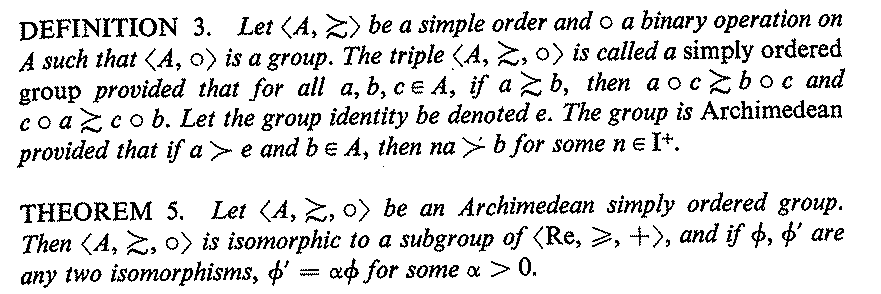Measurement TheoryLouis Narens web email Kent Johnson web email

Introduction
Why do we use numbers in science to represent empirical phenomena? Why do we use these numbers in some ways rather than others? Broadly speaking, these are the kinds of questions this seminar addresses.

Consider a mundane example. Suppose you're describing two sculptures you recently saw. You wouldn't describe one of them simply as having a height of "2'', without specifying whether this height was in inches, feet, meters, etc. But while comparing their heights, you might say that one of them was "twice'' as tall as the other, without specifying your units of measurement you used.
• Question: What is it about an empirical phenomenon like "lengths" that makes it impossible for us to assign individual numbers to them without specifying our units of measurement?
• This question assumes that such an assignment really is impossible; this assumption is true, in a very strong sense.
• Question: Why can lengths be compared without reference to how they were measured?
• Such comparisons can't be made willy-nilly: the ratio between measurements of lengths doesn't depend on whether we used feet or meters, but the difference (via subtraction) between them does. Why?

Answers to these questions and many others will also help us address some much deeper issues, such as:
• Question: Why do the empirical sciences use only a few different scales of measurement (ratio, interval, etc.)?
• Short answer: Amazingly, the mathematical universe simply doesn't contain very many such scales! (Think in the neighborhood of 3 to 7 possible scales, depending on how you count.)

Unless noted otherwise, references below are to: Krantz, D. H., Luce, R. D., Suppes, P., and Tversky, A. (1971). Foundations of Measurement Volume 1: Additive and Polynomial Representations. Academic Press, Inc., San Diego.

 Week Topic 1 Introduction Optional: [paper] Hand (1996): "Statistics and the theory of measurement" Optional: [paper] Swoyer (1991): "Structural representation and surrogative reasoning"  [Background: Chap. 1 [chapter] Introduction Chap. 2: [chapter] Construction of Numerical Functions Theorems 4, 4': Main isomorphism theorem Theorem 5: Holder's theorem Theorem 2: Order-isomorphism theorem [Theorem 6: counterpoint: absolute scale example] 2 Chap. 2 continued Chap. 3: Extensive Measurement Theorem 1: Closed extensive structures Theorem 3: Extensive structures with no essential maximum Ellis' alternative construction Alternative numerical representations [Theorems 8-9: standard sequences] 3 Chap. 3 continued 4 Chap. 4: Difference Measurement Theorem 1: Positive-difference structures Theorem 2: Algebraic-difference structures [Theorem 3: ratio and difference representations] [Theorem 6: Absolute-difference structures] 5 Chap. 5: Probability Representations Theorem 2: Qualitative Probability Nonsufficiency of Qualitative Probability Luce's Axiom Theorem 4: Countable additivity [Theorem 7: Qualitative Conditional Probability] 6 Chap. 6: Additive Conjoint Measurement Theorem 2: Additive Conjoint Structures [Theorem 13: n-component additive conjoint structures] [Chap. 7, Theorem 1: decomposable structures] [Chap. 9, Section 4: Applications] Optional: [paper] Simonov et al (2014): "On an algebraic definition of laws" 7 [No class: Holiday] 8 Selected Topics Theorem 2 concluded [paper] Narens & Luce (1986): "Measurement: The theory of numerical assignments" 9 Selected Topics [paper] Narens (1981a): "A general theory of ratio scalability with remarks about the measurement-theoretic concept of meaningfulness " Optional: [paper] Narens (1981b): "On the scales of measurement" [Lecture slides] 10 Selected Topics [paper] Narens (1981a) continued (emphasis on sections 1-2) [paper] Narens (1988): "Meaningfulness and the Erlanger program of Felix Klein" Finals Week [paper] Luce & Narens (1992): "Intrinsic Archimedeanness and the continuum"

• Optional: [paper] Luce (1997): "Several unresolved conceptual problems of mathematical psychology"
• Optional: [paper] Luce (1996): "The ongoing debate between empirical science and measurement theory"
• Fun: [exhibition] Archimedes palimpsest at the Huntington Library

Further References
• Hand, David J. (2004). Measurement theory and practice: The world through quantification. London: Hodder.
• Matthews, Robert (2007). The measure of mind: Propositional attitudes and their attribution. Oxford: OUP.
• Narens, Louis (1985). Abstract measurement theory. Cambridge, MA: MIT.
• Narens, Louis (2002). Theories of meaningfulness. Mahwah, NJ: Laurence Erlbaum.
• Narens, Louis (2007). Introduction to the theories of meaningfulness and the use of symmetry in science. Mahwah, NJ: Laurence Erlbaum.
• Suppes, Patrick (2002). Representation and invariance of scientific structures. Stanford: CSLI.
• Trout, J.D. (1998). Measuring the intentional world: Realism, naturalism, and quantitative methods in the behavioral sciences. Oxford: OUP.